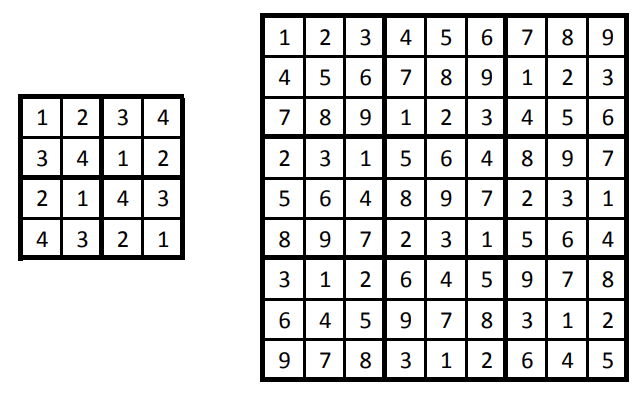시간 제한메모리 제한제출정답맞힌 사람정답 비율
1 초 512 MB28171571.429%

## 문제

Fereydun, the legendary Persian hero whose prophecy was to overcome Zahhak, believes that he needs a powerful mind together with a powerful body. He has just learned a new brain teaser, called Sudoku, from a Japanese trader.

Sudoku is played on a board that is a grid of $n^2 \times n^2$ cells. The whole grid is also partitioned into $n^2$ sub-grids, each of size $n \times n$. Each cell can be empty or contain an integer from $1$ to $n^2$ (inclusive). A Sudoku board is valid if it meets the following conditions:

1. All numbers in each row are distinct.
2. All numbers in each column are distinct.
3. All numbers in each sub-grid are distinct.

The following figure shows two valid Sudoku boards with no empty cells:Fereydun has a valid board with some empty cells and has asked for your help. Your task is to fill as many empty cells as you can while keeping the board valid. It is guaranteed that there is at least one way to fill all the empty cells while keeping the board valid.

This is an output-only problem, so no source code submission is required. There are $10$ test cases. For each test case, you are given an input file containing a table $A$ as the initial state of a Sudoku board, and your task is to submit an output file containing a table $B$ as the final state of that Sudoku board after filling some of its empty cells. All $A[i][j]$ and $B[i][j]$ values (for $1 \leq i,j \leq n^2$) are integers between $0$ and $n^2$ (inclusive), where value $0$ indicates an empty cell.

## 입력

The input is in the following format:

• line $1$: $\;\;n$
• line $1+i$ (for $1 \leq i \leq n^2$): $\;\; A[i] \;\; A[i] \;\ldots \; A[i][n^2]$

## 출력

The output must be in the following format:

• line $i$ (for $1 \leq i \leq n^2$): $\;\; B[i] \;\; B[i] \;\ldots \; B[i][n^2]$

## 제한

• $2 \leq n \leq 20$,
• $0 \leq A[i][j] \leq n^2$,
• The board $A$ is valid, and all of its empty cells can be filled while keeping it valid.

## 점수

Your output for a test case will get $0$ score if $B$ is not a valid board, or some non-empty cell of $A$ has a different value in $B$. Otherwise, the score will be $10 \times (p-q)/p$ where $p$ and $q$ are the number of empty cells in $A$ and $B$, respectively.

It is not necessary that the empty cells of $B$ can be completely filled while keeping the board valid.

## 예제 입력 1

2
0 2 0 0
3 0 0 0
0 0 4 0
0 0 0 1


## 예제 출력 1

4 2 3 0
3 1 2 4
1 3 4 2
2 4 0 1


In this example, the values of $p$ and $q$ are $12$ and $2$, respectively. So, the this output gets $10 \times (12-2)/12 = 8.33$ points.

Text

## 채점 및 기타 정보

• 10점 이상을 획득해야 를 받는다.
• 예제는 채점하지 않는다.